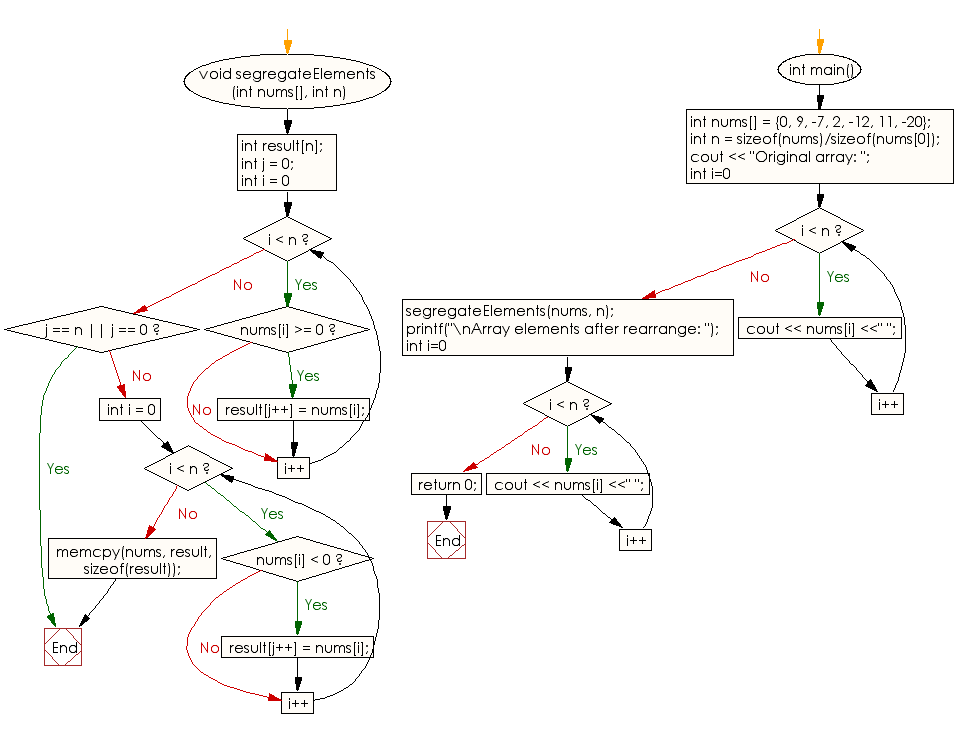﻿ C++ : Move all negative elements at the end of an array# C++ Exercises: Move all negative elements of an array of integers to the end of the array without changing the order of positive element and negative element

## C++ Array: Exercise-18 with Solution

Write a C++ program to move all negative elements of an array of integers to the end of the array without changing the order of positive element and negative element.

Sample Solution:

C++ Code :

``````#include <iostream>
using namespace std;

void segregateElements(int nums[], int n)
{
// Array to store result
int result[n];

int j = 0; // index of result
for (int i = 0; i < n ; i++)
if (nums[i] >= 0 )
result[j++] = nums[i];
if (j == n || j == 0)
return;

for (int i = 0 ; i < n ; i++)
if (nums[i] < 0)
result[j++] = nums[i];

// Copy contents to nums[]
memcpy(nums, result, sizeof(result));
}

int main()
{
int nums[] = {0, 9, -7, 2, -12, 11, -20};
int n = sizeof(nums)/sizeof(nums);
cout << "Original array: ";
for (int i=0; i < n; i++)
cout << nums[i] <<" ";
segregateElements(nums, n);

printf("\nArray elements after rearrange: ");
for (int i=0; i < n; i++)
cout << nums[i] <<" ";
return 0;

}
``````

Sample Output:

```Original array: 0 9 -7 2 -12 11 -20
Array elements after rearrange: 0 9 2 11 -7 -12 -20
```

Flowchart:C++ Code Editor:

What is the difficulty level of this exercise?

Test your Programming skills with w3resource's quiz.

﻿